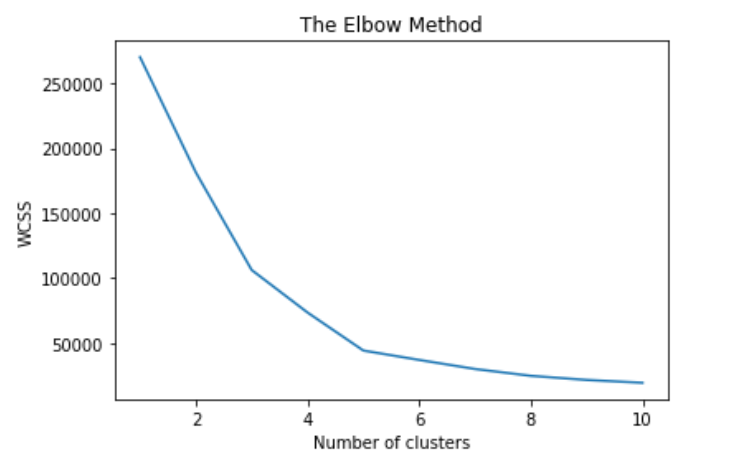# Introduction

## What is Clustering?

Clustering is basically a type of Unsupervised learning method. It is the process of dividing the population into groups consisting of similar data points. Points lying in the same group are as similar as possible and points in different groups are as dissimilar as possible.

## Why Clustering?

Clustering helps in understanding the natural grouping in a dataset. Their purpose is to make sense to partition the data into some group of logical groupings. The main advantage of a clustered solution is automatic recovery from failure, that is, recovery without user intervention.

## Types Of Clustering:

1. Exclusive Clustering: It is considered hard clustering. Here data points/items belong exclusively to one cluster. Example: K-Means Clustering.
2. Over Lapping Clustering: It is considered soft clustering. Here data points/items belong to multiple clusters. Example: Fuzzy/C-Means Clustering.

# K-Means Clustering

## Introduction

K-Means Clustering is a type of unsupervised learning, which is used when you have unlabeled data (i.e., data without a Target Column). The goal of this algorithm is to find groups in the data, with the number of groups represented by the variable K.

## Working

Before applying any algorithm on datasets it’s necessary to understand it’s working so, let’s deep dive into the working of K-Means Clustering:

1. At very first we need to choose the number K of Clusters (Let’s take K=2).

2. Then selecting at random K points, the centroids (not necessary from the dataset).

3. Then assign each data point to the closest centroid → that forms K Cluster.

4. Then it computes and places the new centroid of each cluster.

5. Reassigning each data point to the new closest centroid. If any reassignment took place, then we go to step 4, otherwise, our model is ready.

Note: The Above Process continues until the best cluster is formed

## How to Choose the Right Number of Clusters?

Finding the right number of clusters is the most difficult and important part of the Algorithm. So, Let’s understand how to choose the right number of clusters in K-Means Clustering.

WCSS: Also known as Within-Cluster-Sum of Squared. It is the sum of the squared distance between each point and the centroid in a cluster.

I will try to show step by step what actually happens to the value of WCSS when the number of Clusters increases, Then I will show the best optimal method to find the right Cluster.

As we are able to find that WCSS is decreasing as we are trying to increase the clusters. Our main aim is to decrease the WCSS. The main task of knowing the right number of clusters is the point after which the WCSS decrease becomes almost constant. So instead of using plots, we will analyze it using the Elbow Method.

From the Above Elbow curve, we found that After 3 Clusters the value of WCSS becomes almost constant so the right number of clusters for this set of data points is 3.

Traditional k-means utilize a randomization process for initializing these centroids, poor initialization can lead to increased numbers of required clustering iterations to reach convergence, a greater overall runtime, and a less-efficient algorithm overall.

To solve this issue we have a solution that is the same as K-Means but has an advantage over bad random initialization called K-Means++. K-Means++ is a smart centroid initialization technique and the rest of the algorithm is the same as that of K-Means. The steps to follow for centroid initialization are:

• Pick the first centroid point (C_1) randomly.
• Compute the distance of all points in the dataset from the selected centroid. The distance of x_i point from the farthest centroid can be computed by
`d_i: Distance of x_i point from the farthest centroidm: number of centroids already picked`
• Make the point x_i as the new centroid that is having maximum probability proportional to d_i.
• Repeat the above two steps till you find k-centroids.

## Implementation:

As of now, we have a rough idea of how K-Means Clustering works. So, Let’s implement it.

1. Importing the Libraries:

2. Importing, Reading DataSet, And Creating the Feature Matrix:

CustomerID is 100% Unique so will never contribute to Model Predictions.

We are using just the last two features(Annual Income (k\$)and Spending Score (1–100) ) for clustering because I wanted to show you guys how clusters actually look if I will use all the features then it will become difficult for visualizing the clusters in a 2D plot.

3. Using the Elbow method to find the optimal number of clusters:From the Elbow Method, we can say that 5 is the optimal number of Clusters

4. Training the K-Means model on the dataset:

5. Visualising the clusters:

The source code of this is available on my GitHub repository.

# Summary:

With this, we came to the end of our article on K-Means Clustering. I hope this article will help you in learning K-Means Clustering in a better manner.

For any queries contact me on LinkedIn.

--

--